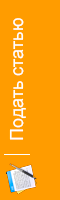Baghinyan M. K. Solving imperfect information game models by applying differential transform method // International scientific journal "Internauka". - 2017. - №12.

Отрасль науки: Технические науки

UDC 004.4:681.5

Baghinyan Mher

National polytechnic university of Armenia

SOLVING IMPERFECT INFORMATION GAME MODELS BY APPLYING DIFFERENTIAL TRANSFORM METHOD

Summary. A method was proposed for solving imperfect information generalized game models based on method of differential transform. An  example of competing advertising companies was considered. Comparative analysis was performed between the acquired results and already known solutions.

Keywords։ imperfect information game model, parametric game model, parametric linear programming problem, parametric simplex method, differential transform.

During the recent years the number of scientific papers and articles dedicated to the games with imperfect (incomplete) information, increased dramatically [1-4].  In  we considered a particular case of such game, where the payoff matrix items are approximate values  and we control the bias of uncertain values by introducing α parameter later in the right side vector of the mathematical programming problem of the equivalent game. We extend the method introduced in  to solve games with incomplete information where the parameters may appear also in payoff matrix.

(1)

Parametric mathematical programming problem in case where the parameters are the constraint coefficients and/or the right side vector, is presented below:

(2)

(3)

where՝  is the matrix of  with parametric coefficients, is the  n-sized vector of unknown variables,  is the parametric vector of the right side constraints.

We apply the same differential - tailor transform method which was introduced in .

where is the tailor image of  original function depending on  real parameter, H is the scale coefficient,  is the approximation center [4-7].

Numerical example։ The game is presented as  two competing companies trying to enhance their market share by introducing new product by means advertisement . Two possible strategies are available for both of them: advertisement by public TV or Newspapers.

We suppose, that the market share of the one company increases while the market share of the other company decreases and also each company puts all its advertisements in one.  The described game is presented by the payoff matrix in table 1, where I  and II companies considered as players I  and II respectively.

Table 1

Payoff matrix of the game

The item (175, 180, 190) in payoff matrix has the following meaning: the sales volume of the first company increases approximately by 180 units, if the  second company also choses the first strategy (TV advertisement).

parameter was introduced for the payoff matrix described in   to get rid of triple elements.

The mathematical programming problem in this case   hase the following form:

(4)

To apply the method of differential transforms we chose the following parameters:  K=2, H=1 and =0 approximation center.

The final results of the game solution for each range of the  parameter, are presented in the table 2 including the function of the game value ,  and  probability functions of the first, second strategies.

Table 2

Final solution results

For  parameter ,  probability functions of the strategies are presented in pic. 1. It is obvious from pic. 1 that function for all the α arguments  in range  has greater value than    function for the same α points. This means,  that for the first company it is profitable to always choose it’s first strategy, which is  TV advertisement.

Pic. 1. ,  probability functions of the strategies in range .

As we see from table 3, the solutions proposed in     has more bias compared to the fixed parameter numerical solutions of the same problem,  than the values of the ,  functions  for the same ranges.   are the values obtained in ,    are the fixed parameter numerical solutions.

Table 3

Comparative analysis table

Conclusion. A new generalized method for solving game models with imperfect information was proposed based on the method of differential transform of G.E. Pukhov. With the application of the proposed method an advertisement incomplete information game was solved. Comparative analysis was performed to check the accuracy of the results.

References

1. Stephanie L. B. Interval Fuzzy Linear Programming Models to Solve Interval-valued Fuzzy Zero-Sum Games / World congress of the international fuzzy systems Association. – Rio Grande, 2015, - p. 16.
2. Seikh M. R., Nayak K. P., Pal M. An alternative approach for solving fuzzy matrix games / International Journal of Mathematics and Soft Computing. – Vol 5.1, 2015, - p. 79-92.
3. Campos L. Fuzzy linear programming models to solve fuzzy matrix games / Fuzzy Sets and Systems, - 1989, Vol. 32, - p. 275–289.
4. Baghinyan M. K. Solving parametric game models by applying differential transform method / Proceedings of NAS RA and NPUA, Series of technical sciences - 2017. - Issue. 70, N 1. – p. 123-130.
5. Baghinyan M. K. A Solution method for none cooperative multi-parametric game models based on differential transforms / «Bulletin NPUA», Series of technical sciences - 2017.- Part 1. - p. 226-232.
6. Пухов Г.Е. Дифференциальные преобразования и математическое моделирование физических процессов. – Киев: Наукова думка, 1986. - 158 с.
7. Симонян С.О., Аветисян А.Г. Прикладная теория дифференциальных преобразований: Монография. - Ереван: Издательство ГИУА "Чартарагет", 2010. - 361с.